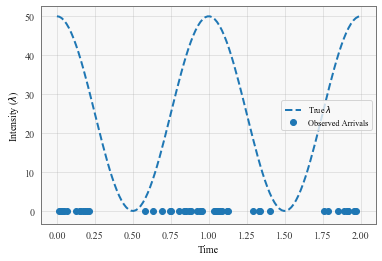[Pyro + GPytorch] Cox Process batching over sequences

Background:

I am trying to model the rate function for my own dataset between 0-24h time window. However, I have several days of data and would like to use one function to capture the variance of all of them.

Question:

I found the Cox process tutorial on the GPytorch website here to be exactly what I was looking for.

However in the example they fit the function base on one sample which they create synthetically at the start of the tutorial.In my case I would have something like this (and would want to train one function for it):

Current hack:

My current solution to this is to just loop over every sequence sample.

for i in range(num_iter):
for sequence in dataset: # this loop not here in tutorial

Question:

1. Even if this is inefficient, is this still addressing the problem correctly? As in, the resultant mean and variances are mathematically correct for my goal?
2. How would you do this properly with batching?

if you really believe that the function is the same for each day you should mod (% in python) all your timestamps by 24 hours and you should be good to go